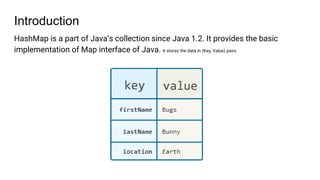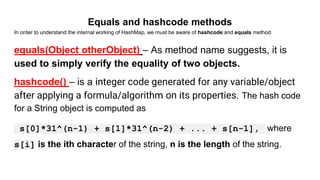Successfully reported this slideshow.

# How Hashmap works internally in java×

# How Hashmap works internally in java

HashMap is a part of Java's collection.. It provides the basic implementation of Map interface of Java. It stores the data in (Key, Value) pairs. To access a value key should be known. This ppt dives deep into the internal working of Hashmaps.

HashMap is a part of Java's collection.. It provides the basic implementation of Map interface of Java. It stores the data in (Key, Value) pairs. To access a value key should be known. This ppt dives deep into the internal working of Hashmaps.

### How Hashmap works internally in java

1. 1. Hashmap Internal Working in Java
2. 2. Introduction HashMap is a part of Java’s collection since Java 1.2. It provides the basic implementation of Map interface of Java. It stores the data in (Key, Value) pairs.
3. 3. Equals and hashcode methods In order to understand the internal working of HashMap, we must be aware of hashcode and equals method. equals(Object otherObject) – As method name suggests, it is used to simply verify the equality of two objects. hashcode() – is a integer code generated for any variable/object after applying a formula/algorithm on its properties. The hash code for a String object is computed as s*31^(n-1) + s*31^(n-2) + ... + s[n-1], where s[i] is the ith character of the string, n is the length of the string.
4. 4. What’s the output? public static void main(String[] args) { String s1 = "Compassites"; String s2 = "Software"; System.out.println( s1.equals(s2) ); System.out.println("Compassites " + s1.hashCode() ); System.out.println("Ea " + s2.hashCode() ); } It Produces the following output. false Compassites hashCode: 1297712907 Software hasCode: 1383974343
5. 5. What about this? public static void main(String[] args) { String s1 = "FB"; String s2 = "Ea"; System.out.println( s1.equals(s2) ); System.out.println("FB hashcode " + s1.hashCode() ); System.out.println("Ea hashcode " + s2.hashCode() ); }
6. 6. What about this? public static void main(String[] args) { String s1 = "FB"; String s2 = "Ea"; System.out.println( s1.equals(s2) ); System.out.println("Compassites " + s1.hashCode() ); System.out.println("Ea " + s2.hashCode() ); } It Produces the following output. false FB hashcode 2236 Ea hashcode 2236 You can see that the hashcodes are same for “FB” and “Ea”. So we can conclude that...
7. 7. Two Rules: 1. If two objects are equal then they must have the same hashcode. (hascode of “aa” and “aa” are same) 2. If two objects have the same hashcode, they may or may not be equal. (string “FB” has hashcode 2236 and “Ea” has hashcode 2236 bu they are not equal) To make it 2nd point clear, we can say that “Birthday as HashCode”
8. 8. Now that we are aware of Hashcode and Equals method, let’s learn the internal working of Hashmap
9. 9. Hashing? HashMap is known as HashMap because it uses a technique called Hashing. Hashing is a technique of converting a large String to small String that represents same String. A shorter value helps in indexing and faster searches.
10. 10. Internally HashMap contains an array of Node and a node is represented as a class which contains 4 fields : It can be seen that node is containing a reference of its own object. So it’s a linked list. Hashmap:
11. 11. Few Terms... Buckets : A bucket is one element of HashMap array. It is used to store nodes. Two or more nodes can have the same bucket. In that case link list structure is used to connect the nodes. Load Factor is a measure, which decides when exactly to increase the hashmap capacity(buckets). HashMap() : It is the default constructor which creates an instance of HashMap with initial capacity 16 and load factor of 0.75. (meaning capacity is doubled when 75% of hashmap is filled) HashMap(int initialCapacity, float loadFactor) : It creates a HashMap instance with specified initial capacity and specified load factor.
12. 12. .
13. 13. Put Operation in HashMap: Say our program is something like this HashMap<String, Integer> map = new HashMap<>(); scores.put (“Rohit”, 140); scores.put (“Dinesh”, 70); scores.put (“Dhoni”, 90); scores.put (“Kholi”, 100); scores.put (“Sachin”, 150); scores.put (“Dravid”, 130); Let’s see what is happening internally...
14. 14. Initially Empty hashMap: Here, the hashmap’s size is 16.(default hashmapsize) HashMap map = new HashMap();
15. 15. Inserting Key-Value Pair: Let us understand at which location below key value pair will be saved into HashMap. scores.put (“Rohit”, 140); When you call the put function then it computes the hash code of the Key. Lets suppose the Hash code of (“Rohit”) is 2657860. Our array has an index till 15, so in order to store “Rohit”, we have to calculate index using a modular operation Index = 2657860 % 16 => 4 Index = 4, So 4 is the computed bucket index value where the entry will sit as a node in the HashMap.
16. 16. Hash Collision Let us understand at which location below key value pair will be saved into HashMap. scores.put (“Dhoni”, 90); When you call the put function then it computes the hash code of the Key. Lets suppose the Hash code of (“Dhoni”) is 63281940. Our array has an index till 15, so in order to store “Rohit”, we have to calculate index using a modular operation Index = 63281940 % 16 => 4
17. 17. Get Operation in HashMap: int rohitScore = scores.get (“Rohit”); So get operation does the same as that of put operation. When the get function is called it basically gets the hash code of the Key. Lets suppose Hash of (“Rohit”) is 2657860 Index = 2657860 % 16 => 4 Now hashMap lookups at bucket index 4 for the hash code of the key “2657860”. Hash code “2657860” found then it lookup for the Key “Rohit” itself in that node or linked list.
18. 18. Thank you :)